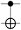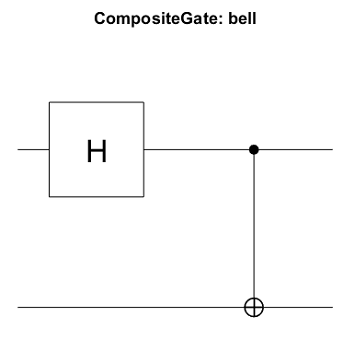# quantumCircuit

Quantum computing circuit

Since R2023a

Installation Required: This functionality requires MATLAB® Support Package for Quantum Computing. Install the support package from Add-On Explorer using the instructions in Get and Manage Add-Ons. Alternatively, see MATLAB Support Package for Quantum Computing on File Exchange.

## Description

A `quantumCircuit` object represents a quantum computing circuit with a specific number of qubits and gates. An example of a quantum circuit with two qubits and two gates is shown in this diagram.Each solid horizontal line in the diagram represents a qubit. The symbolsandrepresent the gates that operate on the qubits. The symbols represent the Hadamard gate and the controlled X gate, respectively. For more details about quantum circuits, see Quantum Circuit.

## Creation

### Syntax

``c = quantumCircuit(numQubits)``
``c = quantumCircuit(gates)``
``c = quantumCircuit(gates,numQubits)``
``c = quantumCircuit(___,Name=name)``

### Description

````c = quantumCircuit(numQubits)` creates a quantum computing circuit with the specified number of qubits and no gates.This syntax sets the NumQubits property to `numQubits`.```

example

````c = quantumCircuit(gates)` creates a quantum computing circuit with the specified quantum gates and the required number of qubits from the gate operations.This syntax sets the Gates property to `gates` and the NumQubits property to the number of automatically created qubits.```
````c = quantumCircuit(gates,numQubits)` creates a quantum computing circuit with the specified quantum gates and number of qubits. The input `numQubits` must be greater than or equal to the largest qubit index used in `gates`. ```

example

````c = quantumCircuit(___,Name=name)` additionally specifies a name for the circuit using any of the input argument combinations in the previous syntaxes.This syntax sets the Name property to `name`.```

### Input Arguments

expand all

Name of the quantum circuit, specified as a string. The `name` input must start with a letter, followed by letters, digits, or underscores (with no white space). The default is an empty string `""`.

When you create a composite gate from an existing circuit using the `compositeGate` function, then this name will also be used as the label for the composite gate (unless you specify a new name when using `compositeGate`). This name will be used in the plot of the composite gate and the function name in the generated QASM code.

Example: `"multi_controlled_Z"`

## Properties

expand all

Number of qubits in the circuit, specified as a nonnegative integer scalar.

Example: `4`

Quantum gates in the circuit, specified as a vector of gates. The array of gates is always returned as a column vector. The elements of this vector must be of type `quantum.gate.SimpleGate` or `quantum.gate.CompositeGate`.

During quantum circuit creation with multiple gates, you can specify `gates` as a row or column vector of gates. However, the `Gates` property always returns a column vector of gates.

Name of the circuit, specified as a string scalar or character vector. This property returns a string scalar. If you do not specify the circuit name when creating the circuit, the default value of this property is an empty string, `""`. Otherwise, the `Name` property value must start with a letter, followed by letters, digits, or underscores (with no white space).

When you construct a composite gate from an existing circuit using the `compositeGate` function, this name is used as the label for the composite gate (unless you specify a new name when using `compositeGate`). This name is also used in the plot of the composite gate and the function name in the generated QASM code.

Example: `"multi_controlled_Z"`

## Object Functions

 `plot` Plot quantum circuit or composite gate `simulate` Simulate quantum circuit `run` Run circuit on quantum device `getMatrix` Matrix representation of quantum circuit or gate `inv` Inverse of quantum circuit or gate `generateQASM` Generate QASM code `unpack` Unpack composite gates in quantum circuit

## Examples

collapse all

Create a quantum circuit that consists of a Hadamard gate and a controlled X gate to entangle two qubits.

```gates = [hGate(1); cxGate(1,2)]; c = quantumCircuit(gates);```

Plot the circuit.

`plot(c)`Create a quantum circuit that consists of Hadamard and controlled NOT gates to entangle two qubits. Name the circuit as `"bell"`.

```innerGates = [hGate(1); cxGate(1,2)]; innerCircuit = quantumCircuit(innerGates,Name="bell")```
```innerCircuit = quantumCircuit with properties: NumQubits: 2 Gates: [2×1 quantum.gate.SimpleGate] Name: "bell"```

Create an outer circuit that contains two composite gates constructed from this inner `"bell"` circuit. The first composite gate acts on qubits 1 and 3 of the outer circuit containing this gate. The second composite gate acts on qubits 2 and 4 of the outer circuit containing this gate.

```outerGates = [compositeGate(innerCircuit,[1 3]) compositeGate(innerCircuit,[2 4])]; outerCircuit = quantumCircuit(outerGates)```
```outerCircuit = quantumCircuit with properties: NumQubits: 4 Gates: [2×1 quantum.gate.CompositeGate] Name: ""```

Plot the outer circuit.

`plot(outerCircuit)`The outer circuit consists of four qubits with indices 1, 2, 3, and 4. The plot shows that qubits 1 and 3 of the outer circuit are mapped to qubits 1 and 2 of the inner circuit of the first composite gate, and qubits 2 and 4 of the outer circuit are mapped to qubits 1 and 2 of the inner circuit of the second composite gate.

Click one of the composite gate blocks in the plot. A new figure showing the internal gates of the composite gate appears.## Version History

Introduced in R2023a